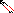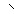# MINLPLib

### A Library of Mixed-Integer and Continuous Nonlinear Programming Instances

#### Instance: ex9_1_8

 Formatsⓘ ams gms lp mod nl osil pip Primal Bounds (infeas ≤ 1e-08)ⓘ -3.25000000 p1 ( gdx sol ) (infeas: 0) Other points (infeas > 1e-08)ⓘ Dual Boundsⓘ -3.25000000 (ANTIGONE)-3.25000000 (BARON)-3.25000000 (COUENNE)-3.25000000 (LINDO)-3.25000000 (SCIP) Referencesⓘ Floudas, C A, Pardalos, Panos M, Adjiman, C S, Esposito, W R, Gumus, Zeynep H, Harding, S T, Klepeis, John L, Meyer, Clifford A, and Schweiger, C A, Handbook of Test Problems in Local and Global Optimization, Kluwer Academic Publishers, 1999.Bard, J F and Falk, J E, An Explicit Solution to the Multi-Level Programming Problem, Computers and Operations Research, 9:1, 1982, 77-100. Sourceⓘ Test Problem ex9.1.8 of Chapter 9 of Floudas e.a. handbook Added to libraryⓘ 31 Jul 2001 Problem typeⓘ QCP #Variablesⓘ 14 #Binary Variablesⓘ 0 #Integer Variablesⓘ 0 #Nonlinear Variablesⓘ 10 #Nonlinear Binary Variablesⓘ 0 #Nonlinear Integer Variablesⓘ 0 Objective Senseⓘ min Objective typeⓘ linear Objective curvatureⓘ linear #Nonzeros in Objectiveⓘ 3 #Nonlinear Nonzeros in Objectiveⓘ 0 #Constraintsⓘ 12 #Linear Constraintsⓘ 7 #Quadratic Constraintsⓘ 5 #Polynomial Constraintsⓘ 0 #Signomial Constraintsⓘ 0 #General Nonlinear Constraintsⓘ 0 Operands in Gen. Nonlin. Functionsⓘ Constraints curvatureⓘ indefinite #Nonzeros in Jacobianⓘ 29 #Nonlinear Nonzeros in Jacobianⓘ 10 #Nonzeros in (Upper-Left) Hessian of Lagrangianⓘ 10 #Nonzeros in Diagonal of Hessian of Lagrangianⓘ 0 #Blocks in Hessian of Lagrangianⓘ 5 Minimal blocksize in Hessian of Lagrangianⓘ 2 Maximal blocksize in Hessian of Lagrangianⓘ 2 Average blocksize in Hessian of Lagrangianⓘ 2.0 #Semicontinuitiesⓘ 0 #Nonlinear Semicontinuitiesⓘ 0 #SOS type 1ⓘ 0 #SOS type 2ⓘ 0 Infeasibility of initial pointⓘ 4 Sparsity JacobianⓘSparsity Hessian of Lagrangianⓘ```\$offlisting
*
*  Equation counts
*      Total        E        G        L        N        X        C        B
*         13       12        0        1        0        0        0        0
*
*  Variable counts
*                   x        b        i      s1s      s2s       sc       si
*      Total     cont   binary  integer     sos1     sos2    scont     sint
*         15       15        0        0        0        0        0        0
*  FX      0
*
*  Nonzero counts
*      Total    const       NL      DLL
*         33       23       10        0
*
*  Solve m using NLP minimizing objvar;

Variables  objvar,x2,x3,x4,x5,x6,x7,x8,x9,x10,x11,x12,x13,x14,x15;

Positive Variables  x2,x3,x4,x5,x6,x7,x8,x9,x10,x11,x12,x13,x14,x15;

Equations  e1,e2,e3,e4,e5,e6,e7,e8,e9,e10,e11,e12,e13;

e1..  - objvar - 2*x2 + x3 + 0.5*x4 =E= 0;

e2..    x2 + x3 =L= 2;

e3..  - 2*x2 + x4 - x5 + x6 =E= -2.5;

e4..    x2 - 3*x3 + x5 + x7 =E= 2;

e5..  - x4 + x8 =E= 0;

e6..  - x5 + x9 =E= 0;

e7.. x11*x6 =E= 0;

e8.. x12*x7 =E= 0;

e9.. x13*x8 =E= 0;

e10.. x14*x9 =E= 0;

e11.. x15*x10 =E= 0;

e12..    x11 - x13 =E= 4;

e13..    x11 + x12 - x14 =E= -1;

Model m / all /;

m.limrow=0; m.limcol=0;
m.tolproj=0.0;

\$if NOT '%gams.u1%' == '' \$include '%gams.u1%'

\$if not set NLP \$set NLP NLP
Solve m using %NLP% minimizing objvar;

```

Last updated: 2021-07-09 Git hash: 827f1a2d